# Variables in C#

## Variables in C# with Examples

In this article, I am going to discuss Variables in C# with Examples. Please read our previous article, where we discussed Type Casting in C# with Examples. At the end of this article, you will understand why we need variables, what is exactly a variable and what the different kinds of variables we can create inside a class what are the role and responsibilities of each type of variable?

##### Understanding Variables in C# Language:

Why we are executing a program means to process the information or data. For example, a bank application. They are executing one program or one transaction. While executing the transaction, what they are actually doing is, they are processing the data like processing the account number, account name, balance, etc.

Whenever we are processing the data or information, the data or information must be at some location. And we call that location a Memory Location. Every computer has memory locations, and every memory location is identified by an address. Just consider in a movie hall, the seating arrangement as memory locations.

So, every memory location in the computer is identified by an address. For a better understanding, please have a look at the below image. As you can see in the below image, 128, 572, 1024, 5098, etc. are one-one memory addresses. We can treat all the addresses are positive integer values.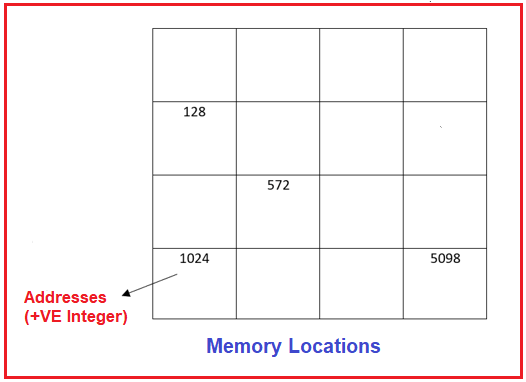##### What is the relation between variable and memory locations?

Suppose I want to store a value of 10 in the computer memory locations. Just consider a classroom, there is no restriction on the students where they can sit. That means the students will go and sit randomly at different locations. In the same way, the value 10 that we want to store in the computer memory locations will also go and be stored randomly at a particular memory location. For a better understanding, please have a look at the below image.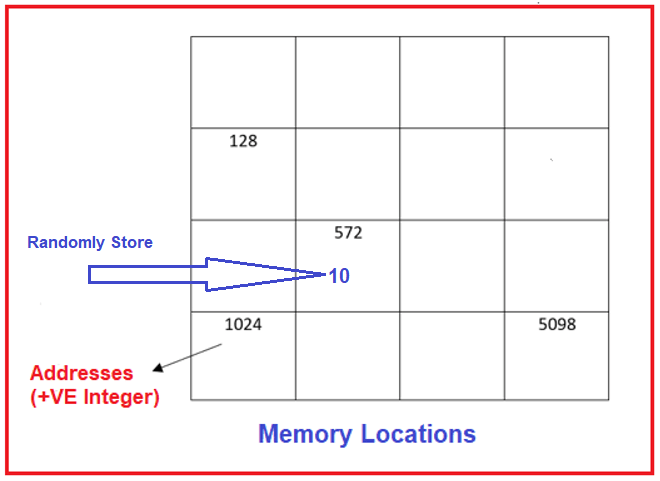##### How to Access the Data?

Now, I want to access the data i.e. value 10, and I just want to print that information. Then how can we print? How we can print the data means we will face problems. The reason is, in which memory locations the data has been stored that we cannot identify because that data is stored randomly at a particular memory location. So, here accessing the memory location becomes very difficult after storing the information. So, what we should do before storing the information, you need to set the identity to the memory location where the data is going to be stored.

##### How we can set Identity to Memory Locations?

We can set the identity of the memory location by using variables or you can say identifiers. The following is the syntax to declare a variable by setting the identity of the memory location in the C# language. First, we need to write the data type followed by the identifier.

Syntax: data_type Identifier;

Example: int a; //Here int is the data type and the identifier can be any name and here we set it as a. So, whenever we declare a variable, it gets memory allocated. To one memory location, the identity is set as shown in the below image.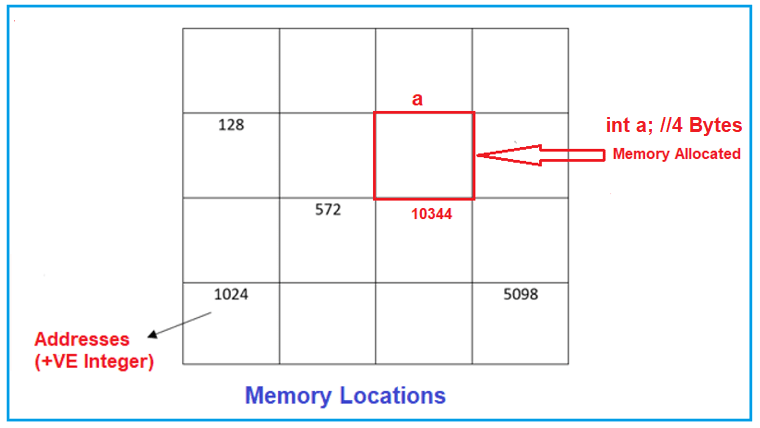Here “a” is a named memory location to location 10344. Later we can store an element in that memory location that is identified by the identifier “a” as follows.

a = 10; //Here, the value is 10 and we are setting this value into a memory location which is identified by “a” as shown in the below image.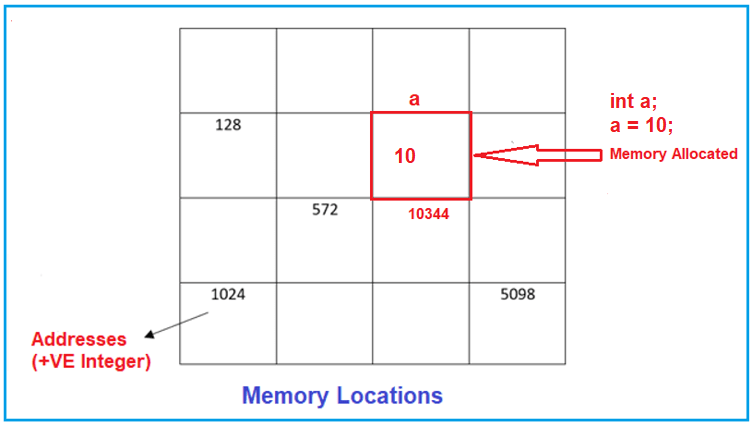For example, in theatres, every seat is having some unique number and when you are coming you will sit in a particular seat that is allocated to you. Later if they want to access it, easily they can access it.

##### What is a Variable in C# Language?

A name that is given for any computer memory location is called a variable. The purpose of the variable is to provide some name to a memory location where we store some data. The user will access the data by the variable name and the compiler will access the data by the memory address. So, the variable is a named location in the computer memory where a program can store the data.

##### Rules for variable declaration in C#:
1. A variable name must begin with a letter or underscore.
2. Variables in C# are case sensitive
3. They can be constructed with digits and letters.
4. No special symbols are allowed other than underscores.
5. sum, Height, _value, and abc123, etc. are some examples of the variable name
##### How to declare a variable in C#?

The Syntax for declaring a variable in C# is as follows:
Syntax: data_type variable_name;
Here, data_type is the type of data to be stored in the variable, and variable_name is the name given to that variable.

Example: int age;
Here, the data type is int and age is the name of the variable where the age variable can only hold an integer value.

##### How to initialize a Variable in C#?

The Syntax for initializing a variable in C# is as follows:
Syntax: data_type variable_name = value;
Here, data_type is the type of data to be stored in the variable, variable_name is the name given to the variable and value is the initial value stored in the variable.

Example: int age = 20;
Here, int is the data type and age is the name of the variable where 20 is the integer value stored inside the age variable.

##### Types of Variables in a Class in C#:

Now, let us understand the different kinds of variables a class can have and their behavior. Basically, there are four types of variables that we can declare inside a class in C#. They are as follows:

1. Non-Static/Instance Variable
2. Static Variable
3. Constant Variable

The behavior of all these different variables is going to vary. Let us understand each of these variables in C#.

##### Static and Non-Static Variables in C#

If we declare a variable explicitly by using the static modifier, we call it a static variable, and the rest of all are non-static variables. Again, if we declare a variable inside a static block, then also that variable is a static variable. And if we declare a variable inside a non-static block, then that becomes a non-static variable.

For a better understanding, please have a look at the following example. In the below example, we have declared three variables. The variable x is a static variable as it is declared using the static modifier. The variable y is non-static by default and the variable z is static as it is declared inside a static block. As the Main method is a static method and hence the variables declared inside the Main method are also going to be static.

```using System;
namespace TypesOfVariables
{
internal class Program
{
static int x; //Static Variable
int y; //Non-Static or Instance Variable
static void Main(string[] args)
{
int z; //Static Variable
}
}
}
```

Now, let us try to print the value of x and y inside the Main method. Let us initialize the x value to 100 and the y value to 200. Here, you can print the value of x directly inside the Main method. But you cannot print the value of y directly inside the Main method.

```using System;
namespace TypesOfVariables
{
internal class Program
{
static int x = 100; //Static Variable
int y = 200; //Non-Static or Instance Variable
static void Main(string[] args)
{
Console.WriteLine(\$"x value: {x}");
}
}
}
```

Output: x value: 100

Now, let us try to print the y value also directly. If we try to print the y value directly, then we will get a compile-time error saying an object reference is required for the non-static field, method, or property ‘Program.y’. For a better understanding, please have a look at the following example. Here, we are trying to print the x and y values directly.

```using System;
namespace TypesOfVariables
{
internal class Program
{
static int x = 100; //Static Variable
int y = 200; //Non-Static or Instance Variable
static void Main(string[] args)
{
Console.WriteLine(\$"x value: {x}");
Console.WriteLine(\$"x value: {y}");
}
}
}
```

When you try to run the above code, you will get the following Compile Time Error.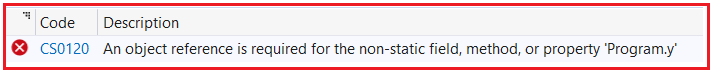This is because the memory for the variable y is going to be created only when we create an instance of the class Program and for each instance. But x does not require an instance of the class. The reason is a static variable is initialized immediately once the execution of the class starts.

So, until and unless we created the instance of the Program class, the memory will not be allocated for the variable y and as long as the memory is not allocated for the variable y, we cannot access it. So, once we create the instance of the Program class, the memory for variable y will be allocated, and then only we can access the variable y.

In the below example, we are creating an instance of the Program class, and using that instance we are accessing the y variable. But we are accessing directly the x variable.

```using System;
namespace TypesOfVariables
{
internal class Program
{
static int x = 100; //Static Variable
int y = 200; //Non-Static or Instance Variable
static void Main(string[] args)
{
Console.WriteLine(\$"x value: {x}");
Program obj = new Program();
Console.WriteLine(\$"y value: {obj.y}");
}
}
}
```

Now, when you run the above code, you will see that it will print both x and y values as shown in the below image.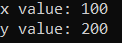Note: The first point that you need to remember is while working with static and non-static variables, static members of a class does not require the instance of a class for initialization and execution also, whereas non-static members of a class require an instance of a class for both initialization and execution.

##### When Static and Non-Static Variables are Initialized in C#?

Static variables of a class are initialized immediately once the execution of the class starts whereas non-static variables or instance variables are initialized only after creating the class instance as well as each and every time the instance of the class is created.

The point we start the execution of a class to the point we end the execution of a class is called a Life Cycle of a class. In the life cycle of a class, static variables are initialized once and only one time whereas non-static or instance variables are initialized 0 times if no instance is created and n times if n instances are created.

Let us understand this with an example. Please have a look at the below code. Here, we are creating the Program class instance two times.

```using System;
namespace TypesOfVariables
{
internal class Program
{
static int x = 100; //Static Variable
int y = 200; //Non-Static or Instance Variable
static void Main(string[] args)
{
Console.WriteLine(\$"x value: {x}");
Program obj1 = new Program();
Program obj2 = new Program();
Console.WriteLine(\$"obj1 y value: {obj1.y}");
Console.WriteLine(\$"obj2 y value: {obj2.y}");
}
}
}
```

In the above example, as soon as the Program execution starts, the memory is allocated for the static variable x. Then we created the instance of the Program class two times, which means the memory is allocated for the y variable twice. Once for the obj1 instance and once for the obj2 instance. For a better understanding, please have a look at the following diagram which represents the memory architecture of the above example.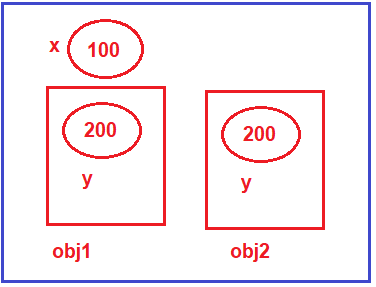As you can see in the above image, the static variable x will be created only once and the non-static variable y will be created two times as we are creating the instance of the Program class two times.

##### Initializing Non-Static variables through Class Constructor in C#:

When we are creating an instance of a class, the constructor call is there and hence we can also initialize the instance variables or non-static variables through the class constructor.

In the previous example, both the objects are having the same y value i.e. 100. Now, if you want then you can provide different values to the y variable using the constructor. Let us understand this with an example. In the below example, we have created one constructor which takes one integer parameter, and this parameter value we are assigning to the non-static y variable. Further, while creating the instance inside the Main method, we are passing different values. Now, whatever value we will pass, that will be going to store inside the non-static y variable.

```using System;
namespace TypesOfVariables
{
internal class Program
{
static int x = 100; //Static Variable
int y = 200; //Non-Static or Instance Variable

//Class Constructor
public Program(int a)
{
y = a;
}

static void Main(string[] args)
{
Console.WriteLine(\$"x value: {x}");
Program obj1 = new Program(300);
Program obj2 = new Program(400);
Console.WriteLine(\$"obj1 y value: {obj1.y}");
Console.WriteLine(\$"obj2 y value: {obj2.y}");
}
}
}
```
###### Output: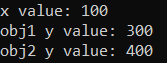Now, in the memory, the value of y for obj1 will be 300 and for obj2 it will be 400. But the x value is going to be the same 100. For a better understanding, please have a look at the below image.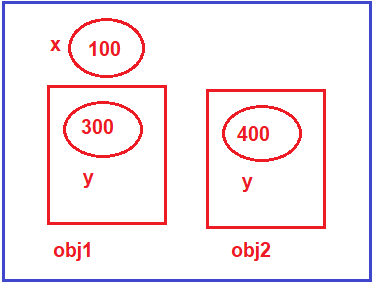Now, you might have one question, can we initialize the static variable through the constructor? The answer is YES. We can initialize the static variable through the constructor. But each time we initialize, the static variable value will override with the new value. For a better understanding, please have a look at the below example. In the below example, we are initializing the static variable through the class constructor. As part of the constructor, we are initializing both the x and y variables with the value of a.

```using System;
namespace TypesOfVariables
{
internal class Program
{
static int x = 100; //Static Variable
int y = 200; //Non-Static or Instance Variable

//Class Constructor
public Program(int a)
{
y = a; //Initializing non-static variable
x = a; //Initializing static variable
}

static void Main(string[] args)
{
Console.WriteLine(\$"x value: {x}"); //x = 100

Program obj1 = new Program(300);
Console.WriteLine(\$"obj1 y value: {obj1.y}");
Console.WriteLine(\$"x value: {x}"); //x = 300

Program obj2 = new Program(400);
Console.WriteLine(\$"obj2 y value: {obj2.y}");
Console.WriteLine(\$"x value: {x}"); //x = 400
}
}
}
```
###### Output: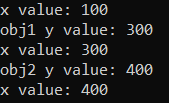For a better understanding, please have a look at the following diagram.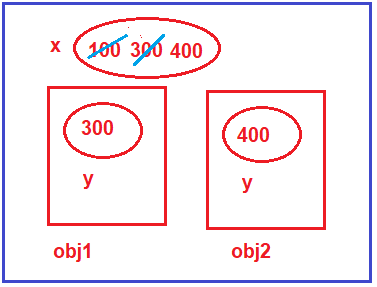So, the point that you need to remember is if you are initializing the static variable through the constructor, then for each constructor execution, it will override the existing value of the static variable. So, in general, we never initialize the static variables through the constructor. If at all, you want to initialize the variable through the constructor, then make that variable non-static.

##### Difference Between Static and Non-Static Variables in C#
1. In the case of an Instance Variable, each object will have its own copy whereas We can only have one copy of a static variable irrespective of how many objects we create.
2. In C#, the Changes made to the instance variable using one object will not be reflected in other objects as each object has its own copy of the instance variable. In the case of static variables, changes made in one object will be reflected in other objects as static variables are common to all objects of a class.
3. We can access the instance variables through object references whereas the Static Variables can be accessed directly by using the class name in C#.
4. In the life cycle of a class, a static variable is initialized only once, whereas instance variables are initialized for 0 times if no instance is created and n times if n number of instances are created.
###### Instance/Non-Static Variables in C#
1. Scope of Instance Variable: Throughout the class except in static methods.
2. The lifetime of Instance Variable: Until the object is available in the memory.
###### Static Variables in C#
1. Scope of the Static Variable: Throughout the class.
2. The Lifetime of Static Variable: Until the end of the program.
##### Constant Variables in C#:

In C#, if we declare a variable by using the const keyword, then it is a constant variable and the value of the constant variable can’t be modified once after its declaration. So, it is mandatory to initialize the constant variable at the time of its declaration only. Suppose, you want to declare a constant PI in your program, then you can declare the constant as follows:

const float PI = 3.14f;

If you are not initializing the const variable at the time of its declaration, then you get a compiler error as shown in the below image.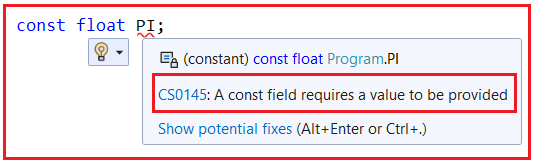As you can see it saying a const field requires a value to be provided which means while declaring a constant it is mandatory to initialize the constant variable.

Note: The constant variables are going to be created once and only one time. This is because we cannot modify the constant values once after its declaration, then if it allows creating multiple copies of the constant variable, then all those copies are going to store the same value which means it is a waste of memory. So, when we cannot modify a value, if we are creating the same copy multiple times, then it is a waste of resources.

The behavior of a constant variable is similar to the behavior of static variables i.e. initialized once and only one time in the life cycle of the class and does not require an instance of a class either for initialization or execution. For a better understanding, please have a look at the following example. The following code is self-explained, so please go through the comment lines.

```using System;
namespace TypesOfVariables
{
internal class Program
{
const float PI = 3.14f; //Constant Variable
static int x = 100; //Static Variable
//We are going to initialize variable y through constructor
int y; //Non-Static or Instance Variable

//Constructor
public Program(int a)
{
//Initializing non-static variable
y = a;
}

static void Main(string[] args)
{
//Accessing the static variable without instance
Console.WriteLine(\$"x value: {x}");
//Accessing the constant variable without instance
Console.WriteLine(\$"PI value: {PI}");

Program obj1 = new Program(300);
Program obj2 = new Program(400);
//Accessing Non-Static variable using instance
Console.WriteLine(\$"obj1 y value: {obj1.y}");
Console.WriteLine(\$"obj2 y value: {obj2.y}");
}
}
}
```
###### Output: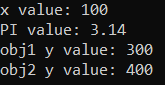The following diagram shows the memory representation of the above example.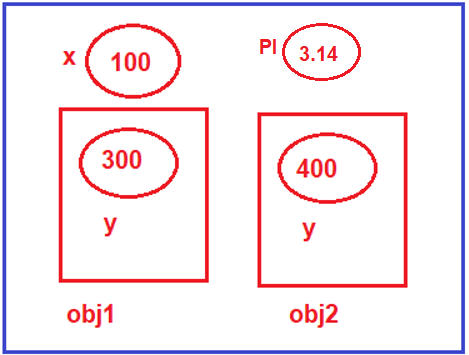Now, you might have one question, if both static and constant are behaving in the same way, then what are the differences between them?

##### Difference Between Static and Constant Variable in C#:

The only difference between a static and constant variable is that the static variables can be modified whereas the constant variables in C# can’t be modified once it is declared. For a better understanding, please have a look at the following example. In the below example, inside the Main method, we are trying the modify both static x and constant PI value.

```using System;
namespace TypesOfVariables
{
internal class Program
{
const float PI = 3.14f; //Constant Variable
static int x = 100; //Static Variable
int y; //Non-Static or Instance Variable

//Constructor
public Program(int a)
{
//Initializing non-static variable
y = a;
}

static void Main(string[] args)
{
//Accessing the static variable without instance
Console.WriteLine(\$"x value: {x}");
//Accessing the constant variable without instance
Console.WriteLine(\$"PI value: {PI}");

x = 700; //Modifying Static Variable
PI = 3.15f; //Trying to Modify the Constant Variable, Error

Program obj1 = new Program(300);
Program obj2 = new Program(400);
//Accessing Non-Static variable using instance
Console.WriteLine(\$"obj1 y value: {obj1.y}");
Console.WriteLine(\$"obj2 y value: {obj2.y}");
}
}
}
```

Now, when you try to run the above code, you will get the following error.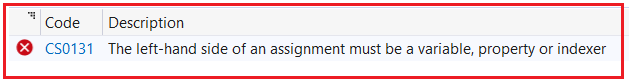As you can see in the above image, it is clearly saying, the left-hand side of an assignment must be a variable, property or indexer. But here, it is a constant and hence we are getting the compilation error.

When we declare a variable by using the readonly keyword, then it is known as a read-only variable and these variables can’t be modified like constants but after initialization. That means it is not mandatory to initialize a read-only variable at the time of its declaration, they can also be initialized under the constructor. That means we can modify the read-only variable value only within a constructor.

The behavior of read-only variables will be similar to the behavior of non-static variables in C#, i.e. initialized only after creating the instance of the class and once for each instance of the class is created. That means we can consider it as a non-static variable and to access readonly variables we need an instance.

##### Example to Understand Read-Only Variables in C#:

In the below example, the read-only variable z is not initialized with any value but when we print the value of the variable, the default value of int i.e. 0 will be displayed.

```using System;
namespace TypesOfVariables
{
internal class Program
{
const float PI = 3.14f; //Constant Variable
static int x = 100; //Static Variable
//We are going to initialize variable y through constructor
int y; //Non-Static or Instance Variable

//Constructor
public Program(int a)
{
//Initializing non-static variable
y = a;
}

static void Main(string[] args)
{
//Accessing the static variable without instance
Console.WriteLine(\$"x value: {x}");
//Accessing the constant variable without instance
Console.WriteLine(\$"PI value: {PI}");

Program obj1 = new Program(300);
Program obj2 = new Program(400);
//Accessing Non-Static variable using instance
Console.WriteLine(\$"obj1 y value: {obj1.y} and Readonly z value: {obj1.z}");
Console.WriteLine(\$"obj2 y value: {obj2.y} and Readonly z value: {obj2.z}");
}
}
}
```
###### Output: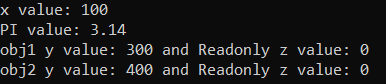In the below example, we are initializing the readonly variable through the class constructor. Now, the constructor takes two parameters. The first parameter will initialize the non-static variable and the second parameter will initialize the readonly variable. So, while creating the instance, we need to pass two integer values to the constructor function.

```using System;
namespace TypesOfVariables
{
internal class Program
{
const float PI = 3.14f; //Constant Variable
static int x = 100; //Static Variable
//We are going to initialize variable y through constructor
int y; //Non-Static or Instance Variable

//Constructor
public Program(int a, int b)
{
//Initializing non-static variable
y = a;
z = b;
}

static void Main(string[] args)
{
//Accessing the static variable without instance
Console.WriteLine(\$"x value: {x}");
//Accessing the constant variable without instance
Console.WriteLine(\$"PI value: {PI}");

Program obj1 = new Program(300, 45);
Program obj2 = new Program(400, 55);
//Accessing Non-Static variable using instance
Console.WriteLine(\$"obj1 y value: {obj1.y} and Readonly z value: {obj1.z}");
Console.WriteLine(\$"obj2 y value: {obj2.y} and Readonly z value: {obj2.z}");
}
}
}
```
###### Output: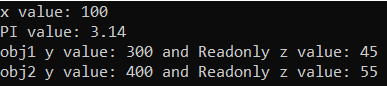For a better understanding of the above example, please have a look at the following diagram which shows the memory representation.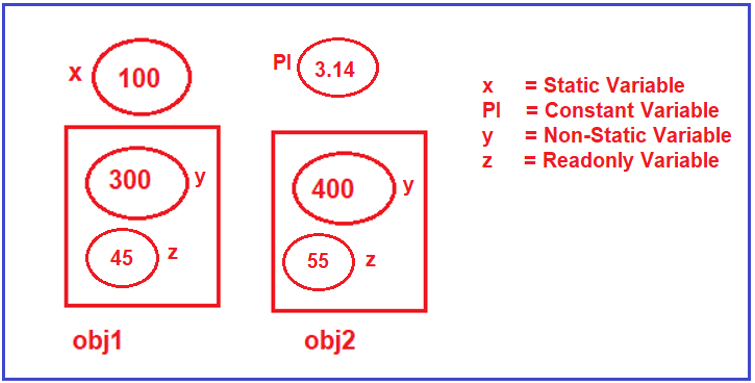Now, again you might have one question, if both non-static and readonly are behaving the same, then what is the differences between them?

##### Difference Between Non-Static and Readonly in C#:

The only difference between a non-static and readonly variable is that after initialization, you can modify the non-static variable value but you cannot modify the readonly variable value. Let us prove this. In the below example, after creating the first instance we are trying to modify the non-static y and readonly z variable value.

```using System;
namespace TypesOfVariables
{
internal class Program
{
const float PI = 3.14f; //Constant Variable
static int x = 100; //Static Variable
//We are going to initialize variable y through constructor
int y; //Non-Static or Instance Variable

//Constructor
public Program(int a, int b)
{
//Initializing non-static variable
y = a;
z = b;
}

static void Main(string[] args)
{
//Accessing the static variable without instance
Console.WriteLine(\$"x value: {x}");
//Accessing the constant variable without instance
Console.WriteLine(\$"PI value: {PI}");

Program obj1 = new Program(300, 45);
//Accessing Non-Static variable using instance
Console.WriteLine(\$"obj1 y value: {obj1.y} and Readonly z value: {obj1.z}");

obj1.y = 500; //Modifying Non-Static Variable
obj1.z = 400; //Trying to Modify Readonly Variable, Getting Error

}
}
}
```

When you try to execute the above code, you will get the following Compilation Error.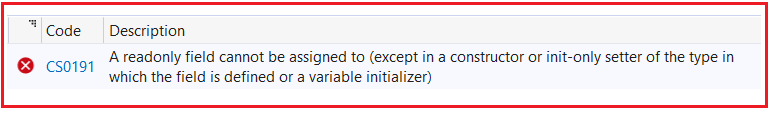As you can see in the above image, it is clearly saying that A readonly field cannot be assigned to (except in a constructor or init-only setter of the type in which the field is defined or a variable initializer). This means you can only initialize a readonly variable at the time of its declaration or through a constructor. And here, we are trying the modify the readonly value inside the Main method and hence we are getting the compilation error.

##### What is the difference between a constant and readonly variable in C#?

The difference between a constant and readonly variable in C# is that a constant is a fixed value for the whole class whereas readonly is a fixed value specific to an instance of a class and for each instance.

##### Local Variables in C#:

The Local Variables in C# are declared inside the method of a class. The scope of the local variable is limited to the method, which means you cannot access it from outside the method. The initialization of the local variable is mandatory.

1. Scope of the Local Variables: Within the block in which it is declared.
2. The lifetime of the Local Variable: Until the control leaves the block in which it is declared.
##### Example to Understand Local Variables in C#:
```using System;
namespace TypesOfVariables
{
internal class Program
{
static void Main(string[] args)
{
}

public void NonStaticBlock()
{
//By Default, every local variable is going to be non-static
//The Scope is limited to this method only
int x = 100;
}

public static void StaticBlock()
{
//By Default, every local variable is going to be static
//The Scope is limited to this method only
int y = 100;
}
}
}

```

1.1.